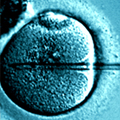### AIMS Bioengineering

2021, Issue 1: 36-51. doi: 10.3934/bioeng.2021005
Research article

# Evaluation of a radial basis function node refinement algorithm applied to bioheat transfer modeling

• Received: 30 October 2020 Accepted: 17 December 2020 Published: 23 December 2020
• Many bioheat transfer problems involve linear/non-linear equations with non-linear or time-dependent boundary conditions. For heat transfer problems, the presence of time and space-dependent functions under Neumann and Mixed type boundary conditions characterize trivial applications in bioengineering, such as thermotherapies, laser surgeries, and burn studies. This greatly increases the complexity of the numerical solution in several problems, requiring fast and accurate numerical solutions. This paper has a main objective evaluate an adaptive mesh refinement radial basis function method strategy for the classical Penne's bioheat transfer modeling. Our numerical results had errors of ~0.1% compared to analytical solutions. Thus, the proposed methodology is accurate and has a low computational cost. For step function heating, two RBF shape parameters were applied, again achieving excellent results. The distributions of the nodes in the solution domain show that the primary source of error in the numerical solutions came from the boundary conditions. This finding should arouse the interest of engineers and scientists in the development of new strategies for problems involving boundary conditions with periodic functions.

Citation: Rafael Pinheiro Amantéa, Robspierre de Carvalho, Luiz Otávio Barbosa. Evaluation of a radial basis function node refinement algorithm applied to bioheat transfer modeling[J]. AIMS Bioengineering, 2021, 8(1): 36-51. doi: 10.3934/bioeng.2021005

### Related Papers:

• Many bioheat transfer problems involve linear/non-linear equations with non-linear or time-dependent boundary conditions. For heat transfer problems, the presence of time and space-dependent functions under Neumann and Mixed type boundary conditions characterize trivial applications in bioengineering, such as thermotherapies, laser surgeries, and burn studies. This greatly increases the complexity of the numerical solution in several problems, requiring fast and accurate numerical solutions. This paper has a main objective evaluate an adaptive mesh refinement radial basis function method strategy for the classical Penne's bioheat transfer modeling. Our numerical results had errors of ~0.1% compared to analytical solutions. Thus, the proposed methodology is accurate and has a low computational cost. For step function heating, two RBF shape parameters were applied, again achieving excellent results. The distributions of the nodes in the solution domain show that the primary source of error in the numerical solutions came from the boundary conditions. This finding should arouse the interest of engineers and scientists in the development of new strategies for problems involving boundary conditions with periodic functions.

Nomenclature

 Symbol Description Unit θc Coarse node parameter - θr Refine node parameter - ωb Blood perfusion m3/s/m3 Ø Radial basis function - Δt Numerical time step s c Specific heat of tissue J/(kg·K) cb Specific heat of blood J/(kg·K) h0 Heat convection coeficient W/(m2·K) k Thermal conductivity of tissue W/(m·K) L Distance between skin surface and body core m Qm Metabolic rate of tissue W/m3 Qr Spatial heating W/m3 r Euclidean distance - t Time s T Tissue temperature °C T0 Steady state temperature °C Ta Arterial temperature °C Tc Blood temperature °C Tf Fluid temperature °C x Spatial coordinate m α Thermal diffusivity m2/s ϵ Multiquadric shape parameter - λ Radial basis function interpolator - ρ Density of tissue Kg/m3 ρb Density of blood Kg/m3###### 通讯作者: 陈斌, bchen63@163.com
• 1.

沈阳化工大学材料科学与工程学院 沈阳 110142Article outline

## Other Articles By Authors

• On This SiteDownLoad:  Full-Size Img  PowerPoint